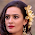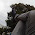VHDL coding tips and tricks: A simple image processing example in VHDL using Xilinx ISE

## Monday, April 27, 2015

### A simple image processing example in VHDL using Xilinx ISE

Unlike with Matlab, where image processing is such a simple task, VHDL can give you few sleepless nights, even for simple tasks. But once you know the basic initial steps, it would become much more easier.

Image processing in VHDL is big topic and its impossible to cover all the areas in a single post. What I try to do here is explain some of the basics with an example.

An image is almost always a 2D matrix. But processing a 2D image in FPGA might not be a good idea. It might lead to excessive delays and resources. So we  convert the 2D image into a linear 1 D array. This data can be stored in a RAM or ROM. To get the most efficient memory module, its recommended that, we use the Block Memory Generator module available in coregen to do this.

In this example, I am going to read the pixels of an image(of size 3*4), stored in a ROM, and store the transpose of the image(of size 4*3) in a RAM.

In brief the steps are:
1. Create a .coe file with the image pixels data.
2. Use coregen in Xilinx ISE to create a simple single port ROM of the required size and load the ROM with the data in steps 1.
3. Use coregen in Xilinx ISE to create a simple single port RAM of the same size as ROM.
4. Write the code where both these RAM and ROM are initiated as components and a process is written to get the transpose of the image stored in ROM.
5. To verify that the RAM contains the correct transposed image, read its contents one by one.
Lets go through the steps in detail now. I have used Xilinx ISE 13.1 for this. The device selected was xc6slx9-2csg324. These steps might be a little different for a different version of Xilinx, but remember that the underlying ideas are still the same.

If you have never used coregen, you might want to go through these examples, before proceeding.

1. Creating .coe file with image pixels:

Open notepad and paste the following text.

memory_initialization_radix=10;
memory_initialization_vector=22,12,200,126,127,128,129,255,10,0,1,98;

Save the file as "bram_data.coe".

2. Create the ROM module:

Look at the screenshots posted below. They should be self explanatory. If a page of settings is missing below, then assume that they remain at their default values.

Click generate and coregen would create the necessary files for you.

3.Create the RAM module:

Once again look at the screenshots below.

4. VHDL code:

This code initiates the RAM and ROM created above and calculates the transpose of the input image. The code also acts as a testbench and reads the data from RAM, to verify the working of the design.
Its not synthesisable because I have incorporated the functionalites of a testbench into this. But if you remove the testbench part its synthesisable.

The code is self explanatory with line by line comments.

library IEEE;
use IEEE.STD_LOGIC_1164.ALL;
use IEEE.STD_LOGIC_ARITH.ALL;
use IEEE.STD_LOGIC_UNSIGNED.ALL;

entity image_process is
end image_process;

architecture Behavioral of image_process is

COMPONENT image1
PORT (
clka : IN STD_LOGIC;
addra : IN STD_LOGIC_VECTOR(3 DOWNTO 0);
douta : OUT STD_LOGIC_VECTOR(7 DOWNTO 0)
);
END COMPONENT;

COMPONENT image2
PORT (
clka : IN STD_LOGIC;
wea : IN STD_LOGIC_VECTOR(0 DOWNTO 0);
addra : IN STD_LOGIC_VECTOR(3 DOWNTO 0);
dina : IN STD_LOGIC_VECTOR(7 DOWNTO 0);
douta : OUT STD_LOGIC_VECTOR(7 DOWNTO 0)
);
END COMPONENT;

signal done,clk : std_logic := '0';
signal wr_enable : STD_LOGIC_VECTOR(0 DOWNTO 0) := "0";
signal addr_rom,addr_ram : STD_LOGIC_VECTOR(3 DOWNTO 0) := (others => '0');
signal data_rom,data_in_ram,data_out_ram : STD_LOGIC_VECTOR(7 DOWNTO 0) := (others => '0');
signal row_index,col_index : integer := 0;

begin

--the original image of size 3*4 stored here in rom.
--[22,12,200,126,
--127,128,129,255,
--10,0,1,98]
image_rom : image1 port map(Clk,addr_rom,data_rom);
--the transpose of image1, of size 4*3, is stored here in ram.
--[22,127,10,
--12,128,0,
--200,129,1,
--126,255,98]
image_ram : image2 port map(Clk,wr_enable,addr_ram,data_in_ram,data_out_ram);

--generate the clock.
clk <= not clk after 5 ns;

--transpose the image1 into image2.
--To do this I have to store the pixel at location (a,b) into location (b,a).
process(clk)
begin
if(falling_edge(clk)) then
if(done = '0') then
addr_rom <= addr_rom + "0001"; --start reading each pixel from rom

--row and column index of the image.
if(col_index = 3) then  --check if last column has reached
col_index <= 0; --reset it to zero.
if(row_index = 2) then --check if last row has reached.
row_index <= 0; --reset it to zero
done <= '1'; --the processing is done.
else
row_index <= row_index + 1; --increment row index.
end if;
else
col_index <= col_index + 1; --increment column index.
end if;

wr_enable <= "1"; --write enable for the RAM
data_in_ram <= data_rom; --store the current read data from rom into ram.
addr_ram <= conv_std_logic_vector((col_index*3 + row_index),4); --set the address for RAM.
else
--this segment reads the transposed image(data written into RAM).
wr_enable <= "0";  --after processing write enable is disabled
addr_rom <= "0000";
if(addr_ram = "1011") then
addr_ram <= "0000";
else
addr_ram <= addr_ram + 1;
end if;
end if;
end if;
end process;

end Behavioral;

5. Simulated waveform:

The design was simulated using Xilinx ISIM. The waveform should look like the following:

#### 3 comments:

1.The above code seems a combination of two separate .veo file. Could you please explain it?

2.Hello,
ROM and RAM was created in this example, created by Xilinx Core generator, but I cant understand where are used ours single port RAM and single port ROM in these code?

3.Can i get a code for removal of impulse noise ,implementation on fpga